1. Modeling
2. Build Models

The Add Version Formula option, on the Edit menu in Blueprint, enables you to specify which versions a formula for a line item subset applies to.

Although the formula will apply across the subset of line items for the version chosen; it won't apply to other modules, even where the line item subset spans several modules.

Once a version formula is set up, it is visible in the formula bar in the module grid but can only be edited in Blueprint.

To apply a formula, each line item must:

• have a version applied.
• belong to a line item subset.
• be configured with the format Number (fields will be blank for data with any other format).

1. Open the module in Blueprint.
3. Select both the line item subset and version that the formula will apply to and click OK.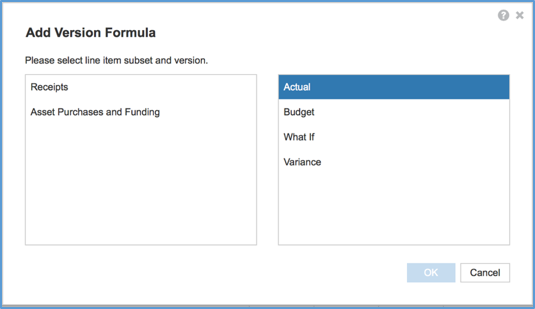4. Enter the formula into the Version Formulae bar.

## Add a version formula for a line item subset

This type of formula can apply to several line items at once for a given version. The formula scope is restricted to a single module.

1. To create the line item subset, under Model Settings > Lists and Roll-ups, click Line Item Subset and then Insert.
2. Name the subset — for example, Regional Costs.
3. Click Line Item Subsets and choose a module. You can select Include New Items to automatically expand the subset as items are added later.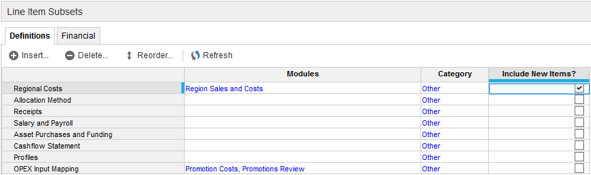4. Select the items to include in the line item subset: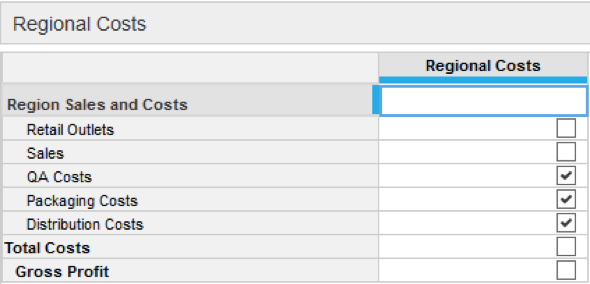5. Open the module and select Blueprint.
6. On the Edit menu, click Add Version Formula and select both the line item subset and the version the formula will apply to.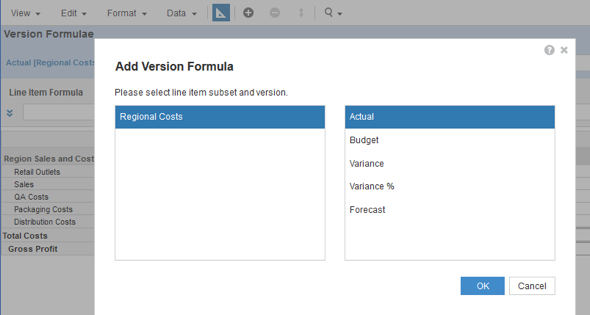If the box on the left of the dialog is empty, it's because the line item subset doesn't contain at least one of the line items in the module.
7. Enter a formula into the Version Formulae bar.
This formula will apply across the subset of line items for the version chosen. Even if the line item subset is common to a number of modules, this formula won't apply to them.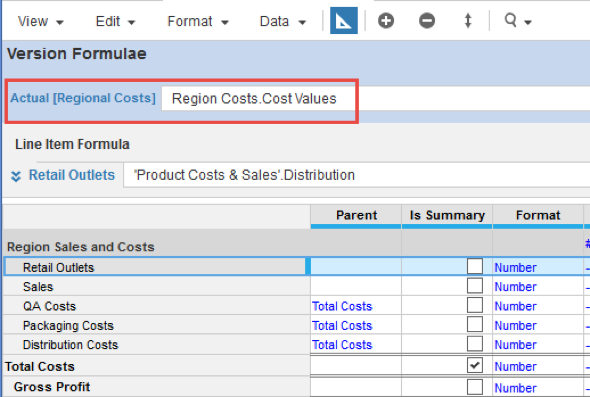This formula can only be edited in Blueprint. In a module grid, if you select a line item to which a version formula applies, you will not be able to edit it in the formula bar.

## Add a version formula for an entire model

This type of formula is typically used for variance calculations and spans the entire model, wherever the Version dimension is applied.

In Model Settings > Versions a version formula can be entered directly into the Formula field.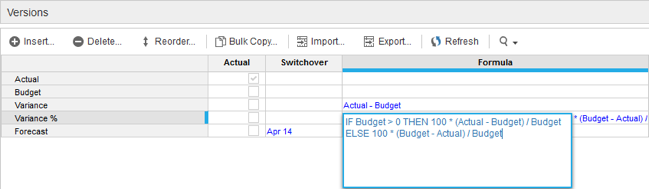Typical version formulas:

Variance = Actual - Budget

Variance % = 100*(IF Budget > 0 THEN 100 * (Actual - Budget) / Budget ELSE 100 * (Budget - Actual) / Budget)